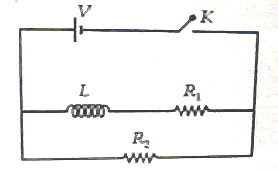## Tuesday, April 27, 2010

### All India Engineering/Architecture Entrance Examination 2010 (AIEEE 2010) Questions Involving Electromagnetic Induction

The following interesting multiple choice questions involving electromagnetic induction were included in the AIEEE 2010 question paper:(1) A rectangular loop has a sliding connector PQ of length l and resistance R Ω and it is moving with a speed v as shown. The set-up is placed in a uniform magnetic field going into the plane of the paper. The three currents I1, I2 and I are

(1) I1 = I2 = Blv/6R, I = Blv/3R

(2) I1 = I2 = Blv/R, I = 2Blv/R

(3) I1 = I2 = Blv/3R, I = 2Blv/3R

(4) I1 = I2 = I = Blv/R

The emf induced in the sliding connector PQ is ε = Blv, which is shown as a battery of internal resistance R in the adjoining figure.

The two external resistances R and R appear in parallel with this battery. The effective external resistance is therefore R/2 and the battery has to drive the current I through a total resistance R + (R/2), which is 3R/2Therefore, I = ε/(3R/2) = 2Blv/3R.

The current I gets divided equally between the two identical branches and hence I1 = I2 = Blv/3R.

(2) In the circuit shown below, the key K is closed at t = 0. The current through the battery is

(1) V(R1 + R2)/R1R2 at t = 0 and V/R2 at t = ∞

(2) VR1R2/√(R12+ R22) at t = 0 and V/R2 at t = ∞

(3) V/R2 at t = 0 and V(R1 + R2)/R1R2 at t =

(4) V/R2 at t = 0 and VR1R2/√(R12+ R22) at t =The current through the inductance branch is zero at the instant the circuit is switched on. [Remember the exponential growth of current in an LR circuit]

Therefore, at the instant t = 0 the current is limited by R2 alone and is equal to V/R2.

At t = ∞ the current has become steady, there is no induced voltage to oppose the flow of current and the inductor functions as a piece of conductor. The current is now limited by R1 and R2 which are in parallel with the battery. The current in this case is V/[R1R2/(R1 + R2)] = V(R1 + R2)/R1R2, as given in option (3).

You will find similar useful multiple choice question (with solution) here.